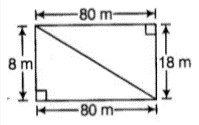# The dimensions of a rectangular`
Question:

The dimensions of a rectangular field are 80m and 18m. Find the length of its diagonal.

Solution:From the question it is given that,

Length of rectangular field = 80 m

Breadth of rectangular field = 18 m

Then,

Length of diagonal = √(length2 + breadth2)

= √(802 + 182)

= √(6400 + 324)

= √6724

= 82 m

∴The Length of diagonal is 82 m.